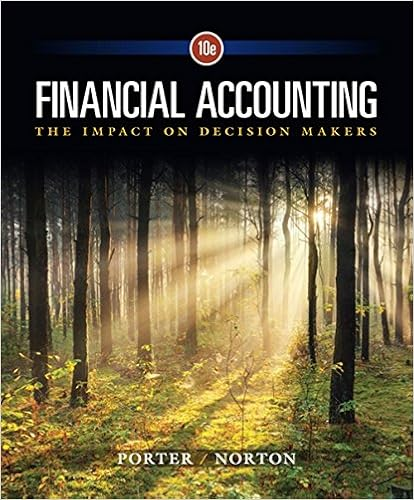# An amount equal to or greater than 8 million and less

• Notes
• 55
• 100% (4) 4 out of 4 people found this document helpful

This preview shows page 4 - 7 out of 55 pages.

##### We have textbook solutions for you!
The document you are viewing contains questions related to this textbook.The document you are viewing contains questions related to this textbook.
Chapter 12 / Exercise 12-22
Financial Accounting: The Impact on Decision Makers
Norton/PorterExpert Verified
A. An amount equal to or greater than \$8 million and less than \$10 millionB. An amount equal to or greater than \$10 million and less than \$12 millionC. An amount equal to or greater than \$12 million and less than \$14 millionD. An amount less than \$6 million or an amount that is equal to or greater than \$14 millionE. The question can not be answered because project D, project E, or both projects (D and E) have cash flows that are not conventional(Spring 2010, quiz 4, question 1)(Fall 2010, quiz 3, question 7)The value created by pursuing a project is its NPV, so the most value that can be created would be the aggregate NPV of all projects with positive NPV.Among projects A, B, and C, projects A and C have positive NPV, so pursuing each of them would increase the amount of value created. Therefore, each should be undertaken. The NPVs of projects D and E must be computed.NPV (D) = [-1.1] + [9.0/1.12] + [7.0/1.122] + [-7.0/1.123] + [-9.0/1.124] = 1.8Project D: npv(12,-1.1,{9.0,7.0,-7.0,-9.0}) \$1.8Project D has a positive NPV, so pursuing it would increase the amount of value created, so it should be undertaken.NPV (E) = [-8.1] + [1.0/1.14] + [2.0/1.142] + [3.0/1.143] + [4.0/1.144] = -1.3Project E: npv(14,-8.1,{1.0,2.0,3.0,4.0}) -\$1.3Project E has a negative NPV, so pursuing it would decrease the amount of value created, so it should not be undertaken.Among all 5 projects, Central Concrete should pursue projects A, C, and DValue created by pursuing projects with positive NPV (in \$ millions) is the sum of their NPVs= 3.1 + 9.7 + 1.8 = 14.6
4
##### We have textbook solutions for you!
The document you are viewing contains questions related to this textbook.The document you are viewing contains questions related to this textbook.
Chapter 12 / Exercise 12-22
Financial Accounting: The Impact on Decision Makers
Norton/PorterExpert Verified
FNAN 301Solutions to test bank problems – capital budgeting criteriaAnswer: D. An amount less than \$6 million or an amount that is equal to or greater than \$14 million5
FNAN 301Solutions to test bank problems – capital budgeting criteria5. The managers of Central Concrete Incorporated are evaluating 5 potential projects (A, B, C, D, and E). Based on the information presented in the 2 tables, what is the maximum amount of value that the managers can create for the firm if they can choose to undertake none, one, some, or all of the 5 potential projects?ProjectInitial investment(in \$ millions)Net present value (in \$ millions)Payback period(in years)Discounted payback period(in years)Internal rate of return(in %)Average accounting return (in %)A1.43.13.64.56.3-5.1B3.2-2.32.121.523.4C4.59.70.84.63.914.5Expected cash flows (number of years from today) in millions of dollarsProjectCost of capital01234D12.0%1.19.07.0-7.0-9.0E14.0%8.11.02.03.04.0
•••